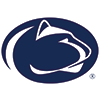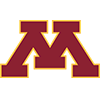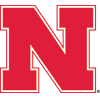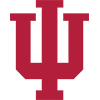DraftKings 2019 Big 10 Odds
-1115
0.09 to 1Ohio State
77.2% implied probability

+800
8 to 1Penn State
9.3% implied probability

+1050
10.5 to 1Wisconsin
7.3% implied probability

+2000
20 to 1Minnesota
4.0% implied probability

+10000
100 to 1Michigan
0.8% implied probability

+20000
200 to 1Nebraska
0.4% implied probability

+25000
250 to 1Iowa
0.3% implied probability

+25000
250 to 1Michigan State
0.3% implied probability

+50000
500 to 1Indiana
0.2% implied probability

+100000
1000 to 1Illinois
0.1% implied probability